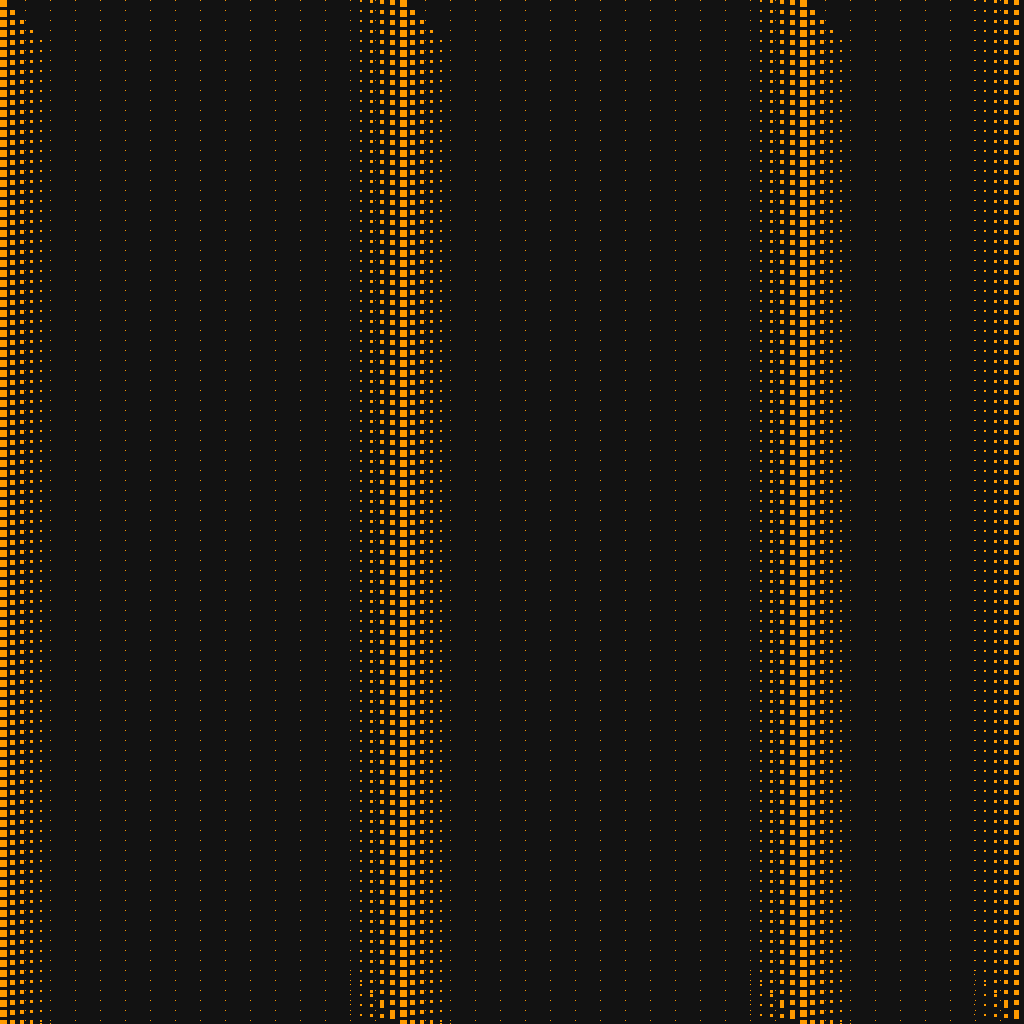Matt Konda

Software meets security meets entrepreneur.

Digital Art Starting Simple

01 September 2021Starting Simple

This post illustrates a super simple piece that starts from a scratch array of black pixels and then programmatically sets some of them, kind of in a wave. Honestly, I just liked the pattern and thought it illustrated some neat things you could do within this construct.

Fields

This was inspired by a painted work that I saw at a gallery in Austin, TX. It was more circular with dots that were circles and which expanded and got smaller as you went in and out from the center of the canvas. Unfortunately, I can find neither the name of the artist or the gallery - which since closed.Code

This is the code I used to create the image. It is interesting how some parts of the process require just tweaking things, like sizes, indexes, offsets. In this case the mods, sizes and colors were things I wanted to mess with as I ran this for fun.

Enjoy!

import numpy as np
from PIL import Image

size = 1024
square = np.empty([size, size, 3], dtype = np.uint8)
color = np.array([255, 155, 0], dtype = np.uint8) # Orange
#color = np.array([255, 255, 255], dtype = np.uint8) # White
square.fill(18) # fill Black

def size_dot(colo, x, y, square, radius):
xi = x + i
yj = y + j
if xi >= size:
xi = size - 1
if yj >= size:
yj = size - 1
square[xi,yj]=colo

for x in range(0, size):
for y in range(0, size):
if y % 25 == 0:
if x % 10 == 0:
square[x,y] = color
if y % 80 == 0:
for i in range (1,6):
size_dot(color, x+(i*10), y+(i*10), square, 6-i)
size_dot(color, x+(i*-10), y+(i*-10), square, 6-i)

size_dot(color, x,y,square,7)

i = Image.fromarray(square)
i.show()